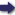(London :  Kegan Paul, Trench, Trübner & Co.,  1910.)

 Tools

## Search this bookPrev Page 43 Next``` CHAPTER LII. 43 Hindus there is no use at all, especially as they require the ctvctma, i.e. the remainder of the partial ilnardtra, for the remainders which we get by the two divisions have two different denominators. He who is perfectlv acquainted with the preceding Rule how to construct a rules of resolution will also be able to carry out the chronoiogi- „ . , ...» . cal date from opposite function, the composition, it a certain amount a certain of past days of a kalpa or caturyitgct be given. To berofdays. make sure, however, we shall now repeat the necessary verse of the ■, ahargana. rules. If we want to find the years, the days being given, the latter must necessarily be civil days, i.e. the differ¬ ence between the lunar days and the ilnardtrct days. This difference [i.e. the civil days) stands in the same relation to their unctrdtrct as the difference between the universal lunar days and the universal ilnctrdtra days, viz. 1,577,916,450,000, to the universal iXnardtra days. The latter number (-i.e. 1,577,916,450,000) is represented by 3,506,481. If we multiply the given days by 55,739, and divide the product by 3,506,481, the quotient repre¬ sents the partial Unardtra days. Adding hereto the civil days, we get the number of lunar days, viz. the sum of the partial solar and the partial adhimdsa days. These lunar days stand in the same relation to the ctdhimdsa days which belong to them as the sum of the uni¬ versal solar and adhimdsa days, viz. 160,299,900,000, to the universal adhimdsa days, which number (t.e. 160,299,900,000) is represented by the number 178,111. If you, further, multiply the partial lunar days by 53II, and divide the product by 178,111, the quotient is the number of the partial adhimdsa days. Subtract¬ ing them from the lunar days, the remainder is the number of solar days. Thereupon you reduce the days to months by dividing them by 30, and the months to years by dividing them by 12. This is what we want to find. E.g. the partial civil days which have elapsed up to ```Prev Page 43 Next